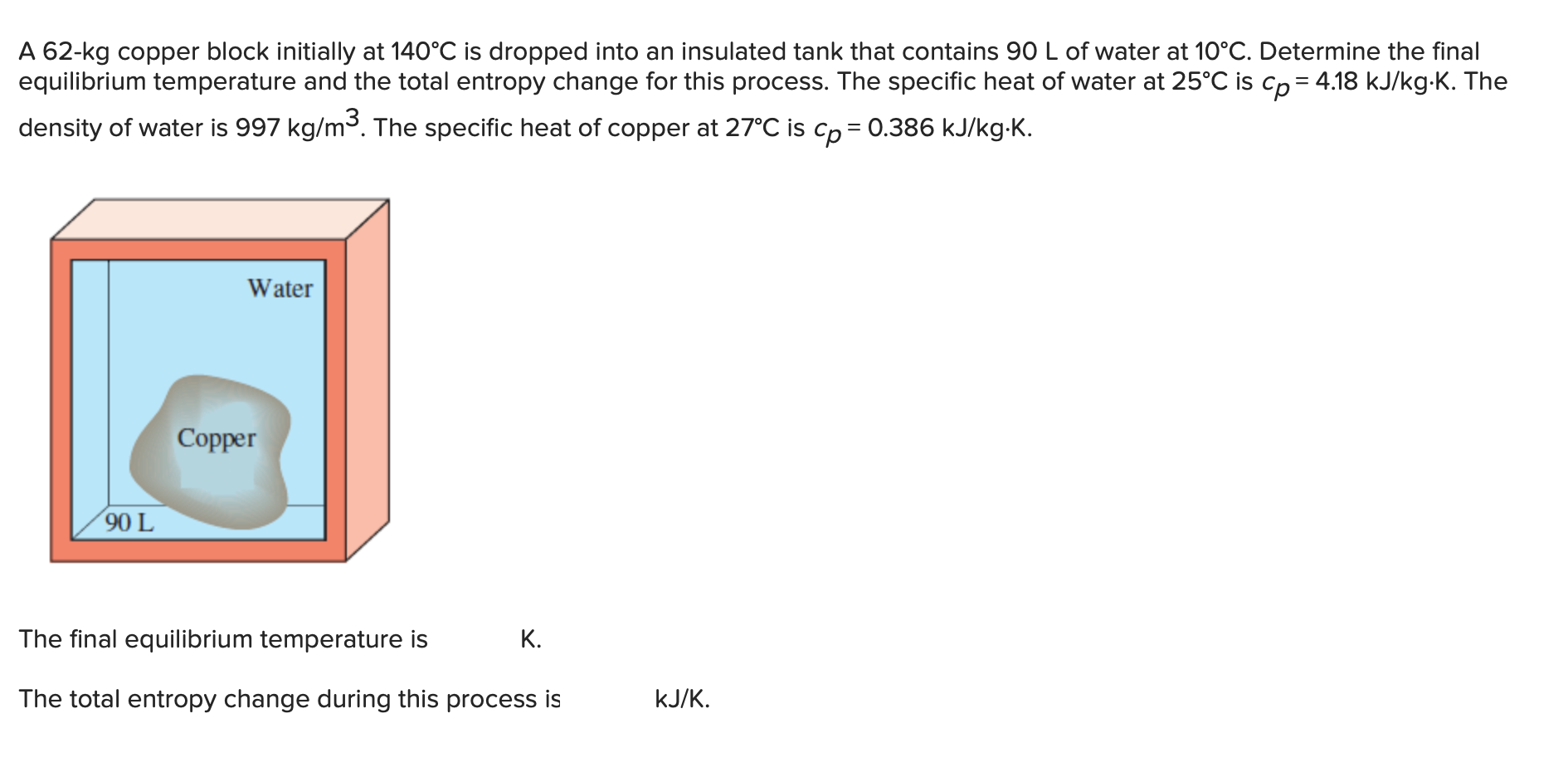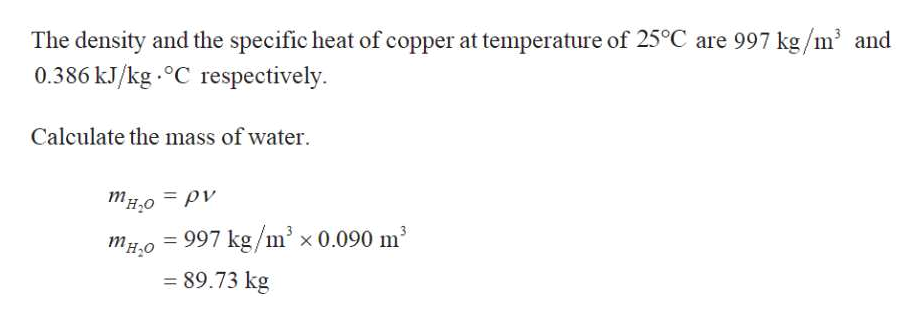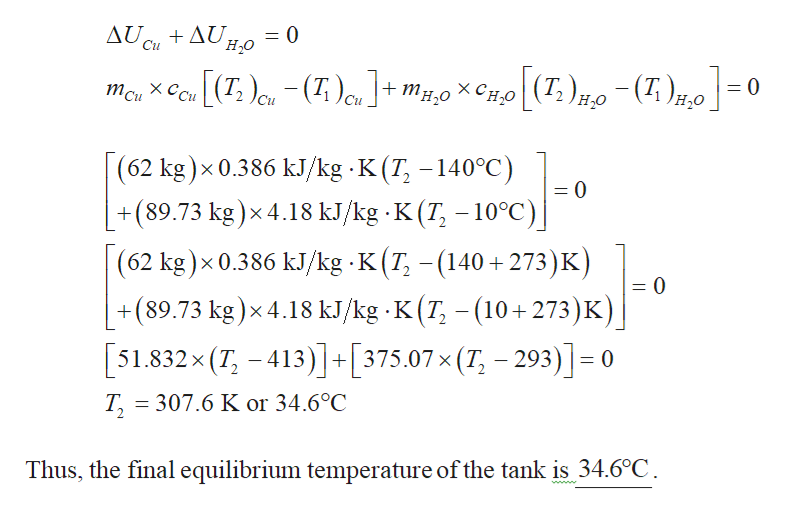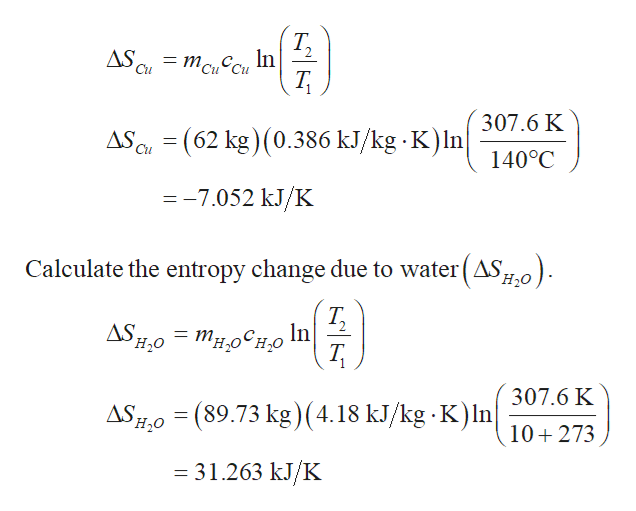# A 62-kg copper block initially at 140°C is dropped into an insulated tank that contains 90 L of water at 10°C. Determine the finalequilibrium temperature and the total entropy change for this process. The specific heat of water at 25°C is cp 4.18 kJ/kg-K. Thedensity of water is 997 kg/m. The specific heat of copper at 27°C is cp0.386 kJ/kg-K.WaterСopper90 LThe final equilibrium temperature isК.kJ/KThe total entropy change during this process is

Question
25 viewshelp_outlineImage TranscriptioncloseA 62-kg copper block initially at 140°C is dropped into an insulated tank that contains 90 L of water at 10°C. Determine the final equilibrium temperature and the total entropy change for this process. The specific heat of water at 25°C is cp 4.18 kJ/kg-K. The density of water is 997 kg/m. The specific heat of copper at 27°C is cp 0.386 kJ/kg-K. Water Сopper 90 L The final equilibrium temperature is К. kJ/K The total entropy change during this process is fullscreen
check_circle

Step 1

Refer the Table “Properties of common liquids, solids, and foods”, select the specific heat of copper  at temperature of 27 degrree celsius as 0.386 kJ/kg.degree.help_outlineImage TranscriptioncloseThe density and the specific heat of copper at temperature of 25°C are 997 kg/m3 and 0.386 kJ/kg.°C respectively. Calculate the mass of water тн,о — ру 997 kg/m x 0.090 m2 тно 89.73 kg fullscreen
Step 2

Write the expression for the total change in internal energy of the system.help_outlineImage TranscriptioncloseAU Cu + Δυ. = 0 н.о Си (T,) H0-(T), хСно - (Т)u+ mно 0 тси Си |(62 kg)x 0.386 kJ/kg K (T, -140°C) 0 +(89.73 kg)x 4.18 kJ/kg K (T -10°C)| 2 (62 kg)x 0.386 kJ/kg K (T (140 +273) K) = 0 +(89.73 kg)x 4.18 kJ/kg K(T -(10+273)K) [51.832x (7, -413)+[375.07x (T, - 293)]- 0 Т, - 307.6 K or 34.6°C Thus, the final equilibrium temperature of the tank is 34.6°C wwww fullscreen
Step 3

Calculate the entropy cha...help_outlineImage TranscriptioncloseТ, In AS = mcuCu Cu 307.6 K AS (62 kg) (0.386 kJ/kg K) In| Cu 140°C =-7.052 kJ/K Calculate the entropy change due to water(AS0 Т, In ASHO о'н,о'ни Т, 307.6 K ASH,O (89.73 kg)(4.18 kJ/kg K)lIn 10+273 = 31.263 kJ/K fullscreen

### Want to see the full answer?

See Solution

#### Want to see this answer and more?

Solutions are written by subject experts who are available 24/7. Questions are typically answered within 1 hour.*

See Solution
*Response times may vary by subject and question.
Tagged in

### Mechanical Engineering